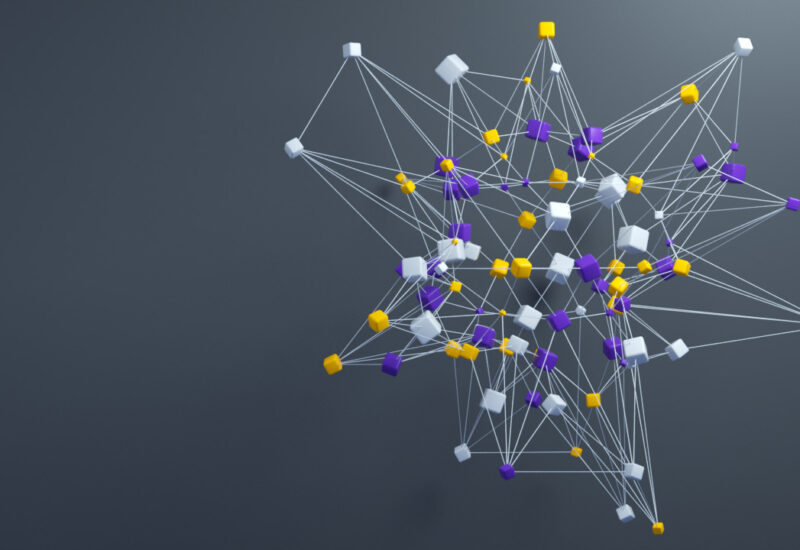# Algorithms (MSR India)

## Algorithms and Data Sciences

Two features are common to much of the field of Algorithms- mathematical guarantees of time and space for even the worst-case input and random access to the entire input. The field however anticipated the explosion of data (“Big data”). Probabilistic Analysis of Algorithms started in the 70’s built models of data and analyzed simple algorithms in the average case. Distributed Computation, Streaming Algorithms and the study of Communication requirements in Algorithms have all received some attention. Data Science, drawing from Statistics and Machine Learning has focused on stochastic models of data and analysis (mainly empirical) of simple algorithms for big data problems. The Algorithms and Data Science research at MSR India brings in the best of all worlds. A focus of ours has been developing mathematical models under which simple algorithms (often ones used widely in practice) have provable guarantees of time and space. Researchers currently at MSR India started the use of sampling from the input to speed up matrix algorithms and this remains one of their interests. Research has also led to better understanding of simple widely used models and algorithms like K-means, gradient descent, alternating minimization, submodular minimization, Topic Modeling and a host of areas. Learning and Optimization areas which have interesting and relevant models and problems.

Some research themes of our focus include:

• Massive Matrix Computation, including randomized and distributed PCA-like problems
• Low rank approximations to Tensors
• Streaming Algorithms
• Property Testing
• Clustering
• Large Scale (distributed, stochastic) Optimization

## Cryptography

Cryptographic algorithms are the core mathematical ingredients of most solutions to security problems. While traditional cryptography focused on securing and authenticating point to point communications, the new world of pervasive connectivity and shared data and computational resources poses new challenges to researchers in cryptography. The last decade has witnessed some fundamental breakthroughs and paradigm shifts in the area of cryptography. These include theoretical breakthroughs that broadly enable computation and access control using encrypted data such as feasibility of Fully Homomorphic Encryption and Functional Encryption. On the other hand, new technologies such as cloud computing also seem to demand practical solutions that combine security and functionality exactly like the ones offered by such theoretical breakthroughs. However, huge gaps remain between the feasibility results from theoretical cryptography and practical demands from emerging technologies. One of the goals of our research is to close these gaps by improving the state of the art on both theoretical aspects and practical applications in this new era of cryptography. We are also actively pursuing alternative methods that combine security and functionality in the context of cloud computing.

More broadly, we focus on the following research directions:

• Attribute based and Functional Encryption and their generalizations
• Composability of protocols
• Nonmalleable Cryptography
• Proofs of Storage
• Secure Multiparty Computation
• Distributed Secure Computation on Large Networks

## Complexity Theory

The primary goal of research in complexity theory may be viewed as understanding the inherent difficulty of problems in terms of the resources algorithms for those problems need. Resources of interest include computational steps (measured by number of bit operations, arithmetic operations, etc.), communication between different parties or parts of a computation, number of random bits needed, and so on. The most challenging problems in complexity theory include proving lower bounds on the complexity of natural problems and hence proving inherent limitations on all conceivable algorithms that solve such problems. The most well-known problem in this area, of course, is the notorious P vs. NP. However, there are interesting problems whose resolutions will signify progress toward the fundamental goals of complexity theory. We are working on several such problems and developing the mathematical tools and techniques that would help attack some of the major challenges in complexity theory.

In particular, some of our focus areas of research include:

• Lower bounds on Arithmetic Complexity, including approaches to the VP vs. VNP problem
• Boolean function complexity, including approaches via Fourier analysis
• Communication Complexity
• Lower bounds on Data Structures

## Theory of Machine Learning

Machine Learning algorithms and optimization techniques have become central to most applications of computing ranging from search, ads, data-mining, data-analytics in large databases, information retrieval and extraction, natural language processing including machine translation, speech, vision, gaming, user adaptation of computing systems, as well as security, privacy, and the broad topic of crowd-sourcing. Our goal is to conduct research in theoretical and practical aspects of Machine Learning and Optimization including:

• Novel machine learning algorithms and paradigms
• Foundational aspects of optimization techniques, including new algorithms and applications to machine learning
• Theoretical analysis of machine learning and optimization algorithms
• Performance analysis and enhancement of machine learning and optimization algorithms
• Applications in search and IR, vision, NLP and other areas
• Data mining and data analytics for very large data sets